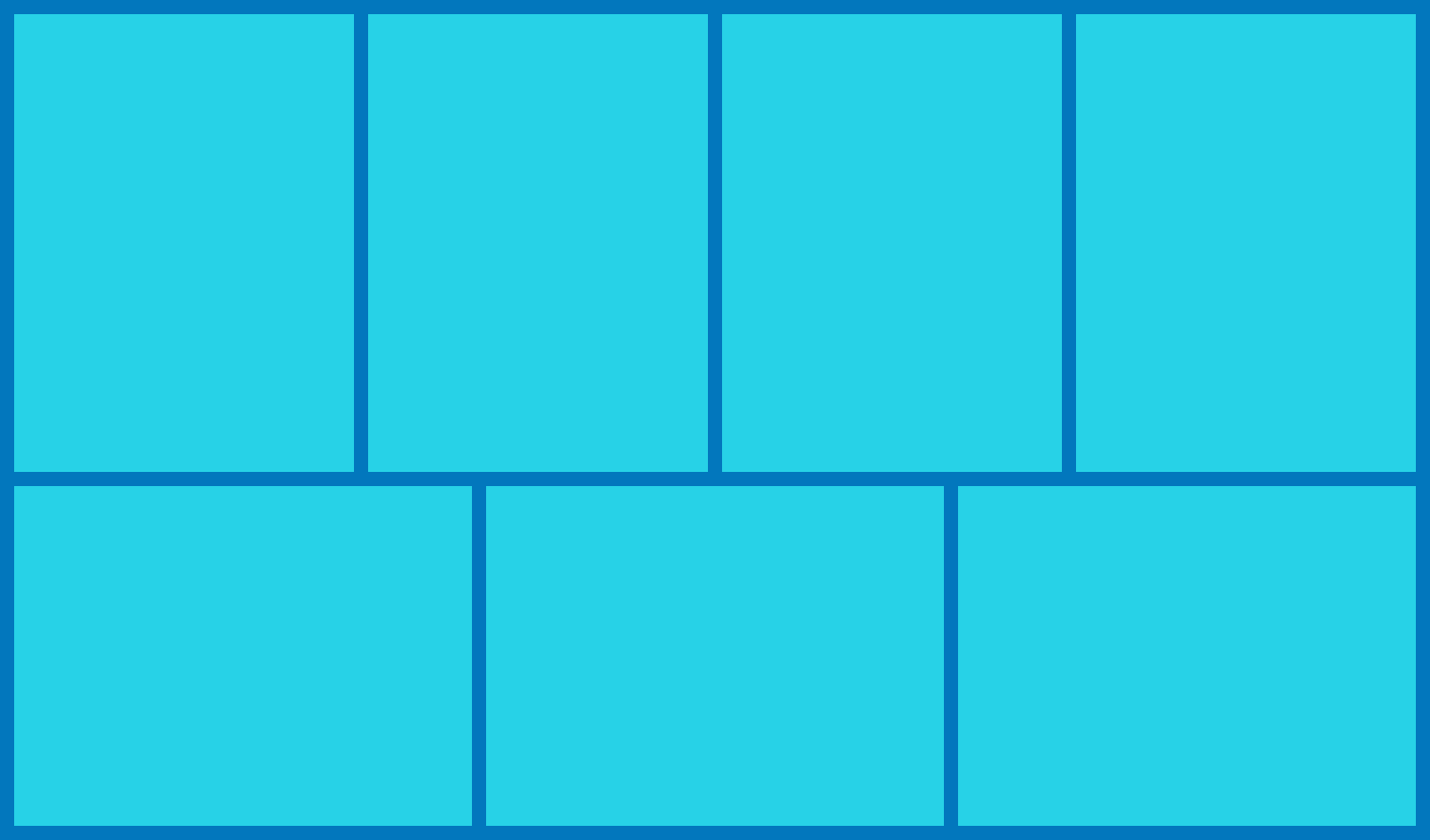# Stacking Rectangles

Geometry Level 2In the given figure, a rectangle of perimeter 76 units is divided into 7 congruent rectangles. What is the perimeter of each smaller rectangle?

×